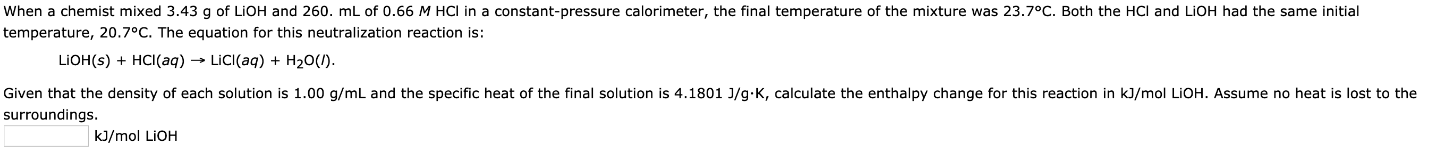# When a chemist mixed 3.43 g of LiOH and 260 mL of 0.66 M HCl in a constant-pressure calorimeter, the final temperature of the mixture was 23.7°C. Both the HCl and LiOH had the same initial temperature, 20.7°C. The equation for this neutralization reaction is: LiOH(s) + HCl(aq) -> LiCl(aq) + H2O(l). Given that the density of each solution is 1.00 g/mL and the specific heat of the final solution is 4.1801 J/g*K, calculate the enthalpy change for this reaction in kJ/mol LiOH. Assume no heat is lost to the surroundings.